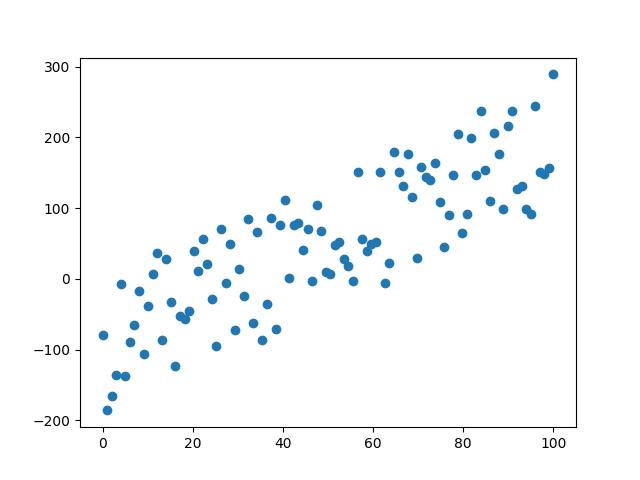# 什麼是梯度下降

在〈什麼是梯度下降〉中留言功能已關閉

# 梯度下降

import pylab as plt
import numpy as np
t=np.linspace(0,10,100)
a=2
v=a*t
S=(1/2)*a*t**2
fig, axes=plt.subplots(1,2, figsize=(12,6))
axes.plot(t, v)
axes.set_title("Speed")
axes.set_xlabel("Time")
axes.set_ylabel("Speed")
axes.plot(t, S)
axes.set_title("Distance")
axes.set_xlabel("Time")
axes.set_ylabel("Distance")
plt.savefig("accele_1.jpg")
plt.show()# 一階微分找極值

$y=x^{2}$ 這個函數要找極值其實很簡單，根本不用什麼逼近法。 只要 y 對 x 作一階微分 $y’=2x$，然後求取 y’=0，也就是說 2x =0，自然就會知道 x=0 時有極值。

# 再度複習迴歸線

import numpy as np
import pylab as plt
n=100
x=np.linspace(0,100,n)
y=3*x+5-np.random.randint(0,100,n)
plt.scatter(x, y)
plt.show()